# If-then-else

Tags

Fill in the blank

Declare a variable

Type the line of code that would declare a variable called `tColorName`, that would hold the name of a color (e.g., purple).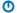Not graded. So why do it?
Multiple choice

What is the string operator & called?A

Append

B

Appendicitis.

C

Concatenation.

D

Cthulhu's appendix.

Not graded. So why do it?

Lesson contents

### The Mighty If statement!

Here's what an If statement is like.

• If test Then
•   As much code as you like
• End If

If `test` is true, then run `As much code as you like`. If `test` is false, skip it. That's the mighty If, and it's what makes software valuable.

Here's an example.

• Dim sAge As Single
• sAge = InputBox("How old are you?")
• If sAge >= 21 Then
•     MsgBox "Welcome! Come on in. Bring your money."
• End If

Let's run it. Type in an age.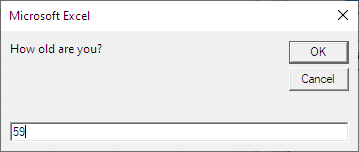Click OK, and see: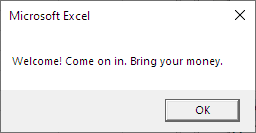A question for you.

Reflect

What happens if the user gives an age of 16?

If you were logged in as a student, the lesson would pause here, and you'd be asked to type in a response. If you want to try that out, ask for an account on this site.Well, I'm guessing that nothing would happen. The program just stops.

You're right.

• Dim sAge As Single
• sAge = InputBox("How old are you?")
• If sAge >= 21 Then If false...
•     MsgBox "Welcome! Come on in. Bring your money."
• End IfRay

Wait. That's not good. The program showing nothing... that could mean anything. Maybe the program crashed.

Hmmm, you're right.

### `If`'s sidekick: `Else`

Mighty `If` can get even mightier.

• If test Then
•   As much code as you like
• Else
•   As much other code as you like
• End If

If `test` is true, then run `As much code as you like`. If `test` is false, run `As much other code as you like`.

Let's improve the code.

• Dim sAge As Single
• sAge = InputBox("How old are you?")
• If sAge >= 21 Then
•     MsgBox "Welcome! Come on in. Bring your money."
• Else
•     MsgBox "Sorry, you're not allowed in yet."
• End If

So...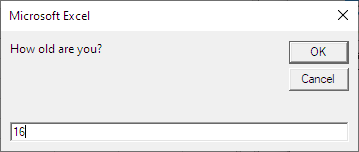... gives...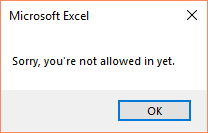One message or the other.

### Indenting

Here's the code again:

• If sAge >= 21 Then
•     MsgBox "Welcome! Come on in. Bring your money."
• Else
•     MsgBox "Sorry, you're not allowed in yet."
• End If

Notice the indenting. Code between `If` and `Else`, and `Else` and `End If`, should be indented four spaces. That helps you remember that the indented statements depend on the `If`. The statements aren't executed, unless the `If` decides they should be.

Press the Tab key to indent a line in VBE.

Indenting your code like this is a requirement for every exercise from now on.

### More Ifs

Here's some more `If` statements, just as examples. Assume we have:

• sAge = InputBox("Goat age (months)?")
• tGoatFaveTVShow = InputBox("Fave TV show?")

Test for old goats.

• If sAge > 120 Then
•     MsgBox "An old goat."
• End If

Heavy goats.

• If sWeight > 40 Then
•     MsgBox "A heavy goat."
• End If

Weightless?

• '<> means not equal to.
• If sWeight <> 0 Then
•     MsgBox "Not a weightless goat."
• End If

Likes Buffy?

• If tGoatFaveTVShow = "Buffy" Then
•     MsgBox "Yes! That's the best TV show ever!"
• End If

All of the tests so far have the form "variable comparison constant," like `sWeight <> 0` and `tGoatFaveTVShow = "Buffy"`. But you can use other expressions on the left and right of the comparison.

For example:

• sSpaceWeight = 0
• If sWeight = sSpaceWeight Then
•     MsgBox "Space goat!"
• End If
• tBestShowEver = "Buffy"
• If tGoatFaveTVShow = tBestShowEver Then
•     MsgBox "Yes! That's the best TV show ever!"
• End If
• sHalfWeightMax = 20
• If sWeight > sHalfWeightMax * 2 Then
•     MsgBox "A heavy goat."
• End If

Notice the calculation in the `If`. `sHalfWeightMax * 2` is computed first, then the comparison to `sWeight` is done.

You could also write it with parentheses, if that would be clearer for you:

• sHalfWeightMax = 20
• If sWeight > (sHalfWeightMax * 2) Then
•     MsgBox "A heavy goat."
• End If

Another:

• sMinMiddleAgeMonths = 36
• If sGoatAgeYears * 12 > sMinMiddleAgeMonths Then
•     MsgBox "An old goat."
• End If

If you prefer:

• sMinMiddleAgeMonths = 36
• If (sGoatAgeYears * 12) > sMinMiddleAgeMonths Then
•     MsgBox "An old goat."
• End If

One more.

• tBestShowEver = "buffy"
• If LCase(tGoatFaveTVShow) = tBestShowEver Then
•     MsgBox "Yes! That's the best TV show ever!"
• End If

Remember that `LCase` converts text to lowercase. So `tGoatFaveTVShow` is converted to lowercase before being compared to `tBestShowEver`. So...

`LCase(tGoatFaveTVShow) = tBestShowEver`

... will be true when `tGoatFaveTVShow` is...

• Buffy
• buffy
• BUFFY
• buFFy
• BuFfY

... or any other strange combination of upper- and lowercase.

However, if `tGoatFaveTVShow` has spaces around it, like " Buffy ", then...

`LCase(tGoatFaveTVShow) = tBestShowEver`

... will not be true. You can allow for the spaces like this:

`Trim(LCase(tGoatFaveTVShow)) = tBestShowEver`

`Trim()` removes leading (on the left) and trailing (on the right) spaces from its parameter (what is inside the `()`).

### More If-Elses

Old goats are cynics.

• If sAge > 120 Then
•     tMessage = "Old goat! Cynical. Depressed."
• Else
•     tMessage = "Goat might still have hope."
• End If
• MsgBox message

Buffy is better.

• If tGoatFaveTVShow = "Buffy" Then
•     tMessage = "Yes! That's the best TV show ever!"
• Else
•     tMessage = "Buffy is better."
• End If
• MsgBox message

### Multiple statements

You can have as much VBA as you like in `If`s and `Else`s. In the next example, each block has two assignments statements.

• 'Multiple statements in an It's statement block.
• If tGoatFaveTVShow = "Buffy" Then
•     tMessage = "Smart, and likes Buffy!"
•     tReward = "Chocolate moose"
• Else
•     tMessage = "Well, we can't all be stars."
•     tReward = "Meat moose"
• End If
• MsgBox message & "vbcrlf" _
•     & "Reward: " & tReward

You can have more. As much code as you want. Including other `If`s (more on that later).

• If tSymbol = "AU" Then
•     sAtomicMass = 196.97
•     tCommonName = "Gold"
•     MsgBox "Me like! Me want!"
• Else
•     MsgBox "OK, that's nice."
• End If

### Fill-in-the-blank

Make sure you get all of these right before going on.

Fill in the blank

Goaty balance

What will `sBalance` be after this runs?

• sBalance = 450
• If sBalance < 500 Then
•   sBalance = sBalance * 2
• End If
• sBalance = sBalance + 100
• If sBalance < 1000 Then
•   sBalance = sBalance - 50
• End IfNot graded. So why do it?
Fill in the blank

Goaty balance returns

What will `sBalance` be after this runs?

• sBalance = 45
• If sBalance > 50 Then
•   sBalance = sBalance / 2
• Else
•   sBalance = sBalance + 5
• End If
• sBalance = sBalance + sAdjust
• If sBalance > 50 Then
• Else
• End If
• sBalance = sBalance - sAdjustNot graded. So why do it?
Fill in the blank

Goaty balance saves Christmas

What will `sBalance` be after this runs?

• sBalance = 100
• If sBalance + sAdjust > 75 Then
•   sBalance = sBalance + 20
• Else
•   sBalance = sBalance - 10
• End If
• sBalance = sBalance + sAdjust
• If sBalance < sAdjust + 40 Then
•   sBalance = sBalance - 50
• End IfNot graded. So why do it?
Fill in the blank

Goaty balance's revenge

What will `sBalance` be after this runs?

• sBalance = 50
• sBalance = sBalance + 20
• sAdjust = sBalance + 10
• If sBalance <> 80 Then
•   sBalance = sBalance + sAdjust * 2
• End IfNot graded. So why do it?
Fill in the blank

The end of goaty balance

What will `sBalance` be after this runs?